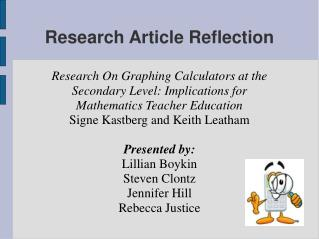DownloadDownload PresentationResearch Article Reflection

# Research Article Reflection

Download Presentation## Research Article Reflection

- - - - - - - - - - - - - - - - - - - - - - - - - - - E N D - - - - - - - - - - - - - - - - - - - - - - - - - - -
##### Presentation Transcript

1. Research On Graphing Calculators at the Secondary Level: Implications for Mathematics Teacher Education Signe Kastberg and Keith Leatham Presented by: Lillian Boykin Steven Clontz Jennifer Hill Rebecca Justice Research Article Reflection

2. Introduction • Graphing calculators were first introduced in 1985 • Teachers soon began exploring how to use these in the classroom. • Key Question: • What does research say about how the use of graphing calculators can impact the teaching and learning of mathematics? • Three main factors: • Access to graphing calculators • Place of calculators in the curriculum • Connection between calculators and pedagogy

3. Access to Calculators • 1994 Survey • 100 middle and secondary mathematics teachers were polled • Around 70% had calculators available for their classroom, but had not used them. • Merely providing teachers with access to graphing calculators does not mean the students have access.

4. What Role Should Graphing Calculators Play in the Classroom? • Teachers usually decide if students should have access to graphing calculators or not based on their own beliefs • Limited access • Associated with belief that students shouldn’t use graphing calculator until they have mastered the certain mathematical procedure • Frequent access • Associated with a belief that graphing calculators should be used to aid in the understanding of a mathematical concept

5. How Does Access to Graphing Calculators Influence Students' Performance? • Study was conducted to compare performance of students with different levels of access to graphing calculators • Twelve classrooms • Assigned to three groups • No access • Access during one unit (about 6 months)‏ • Access for one year

6. How Does Access to Graphing Calculators Influence Students' Performance? • Results • Students with longest access to calculators “tended to attempt more problems and obtain higher test scores than the students who had not” • Also, even limited access to calculators may have a positive result on students’ approaches to mathematical problems

7. Calculators in the Curriculum • Effective use of graphing calculators allows quick and easy development of and translation between representations. • Curriculum developers quickly realized the power of these tools and designed investigations that made use of the device as a lever to learn mathematics.

8. Curricula Designed for Graphing Calculators • CPMP and UCSMP were developed to take advantage of graphing calculators. • Studies found that students taught using these curricula outperformed those taught using traditional approaches on application problems. • However, the findings of the two studies differ with regard to student performance on procedural tasks. • In each of the studies, the authors suggested that the method of instruction was a critical factor in determining student learning.

9. Importance of Pedagogy • The results from a study of the effects of pedagogy found that students’ approaches to various problems revealed that the performance of students in each class was closely aligned with the pedagogy of their teachers. • The authors found that “students ‘behave’ as they are taught” solving problems using algebraic, graphical, or multiple representations depending on the representations their teachers presented most often.

10. Implications • Access • In order for graphing calculators to work effectively, teachers need access to use them • Curriculum • Teachers need to reflect on the pros and cons of incorporating the graphing calculator in the classroom. • Pedagogy • Teachers need to be informed on current research done on graphing calculator. This will allow them to know the ways that other teachers are using them in their curriculum.